# Fixed Area, Infinite Perimeter

The Koch Snowflake (named after its inventor, the Swedish mathematician Helge von Koch) is a fractal with a number of interesting properties.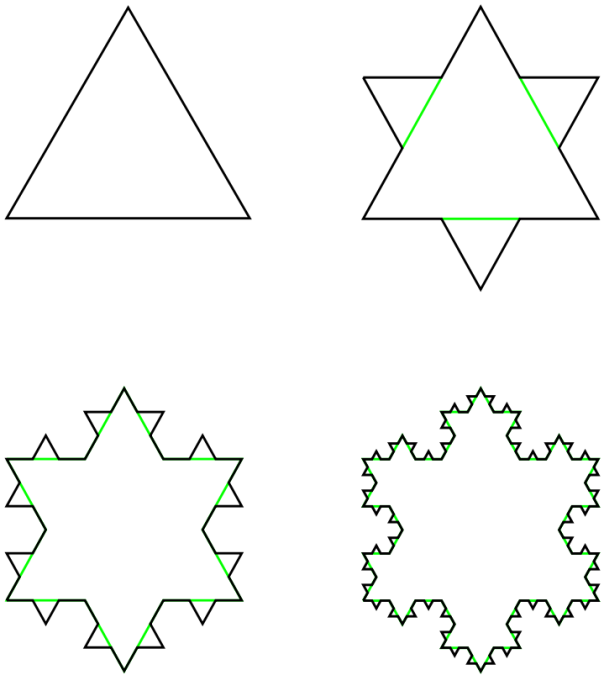The first four generations of the Koch Snowflake

As the number of generations increases, the area of the snowflake increases, but it increases towards a limit: eight-fifths of the size of the first (triangular) generation. This is because each additional generation adds three triangles which are one-ninth the size of the triangle added in the previous generation (for a total increase of one-third), and the additional of increasingly small triangles has a lesser and lesser effect on the overall area as the number of generations increases.

But as the number of generations increases, the perimeter of the shape continues to grow, without approaching a limit: each generation of the Snowflake has a perimeter which is four-thirds of the previous generation’s perimeter. This is because the additional perimeter added each time is four times one-third of the length of each side, for a total increase of four-thirds, as opposed to the one-third increase in area. Because four-thirds is greater than one, the perimeter tends to infinity, whereas the area (which at one-third is less than one) does not.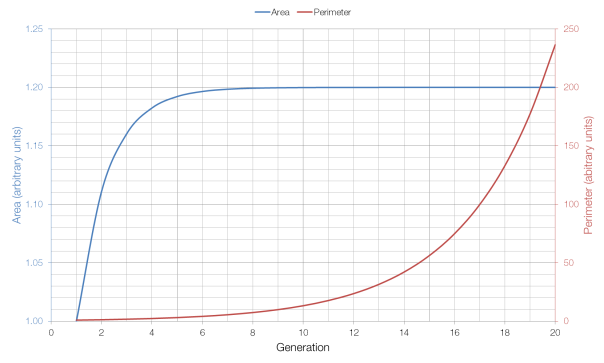As you can see from the graph above, the area approaches its limit very quickly, whereas the perimeter grows very quickly (which is why it has to be shown on a different axis).

# Rectangles and Squares

Just very quickly, to clarify:

• A rectangle is any two-dimensional shape with four right-angled corners.
• A square is a rectangle in which all four sides are the same length.
• Therefore all squares are rectangles, but not all rectangles are squares.

I hope that clears things up.

# How big are pizzas?

Is a 12-inch pizza twice as big as a 6-inch pizza?

Simply put, no. The amount of pizza (its area) is proportional to the square of its diameter, so a 12-inch pizza is actually four times bigger than a 6-inch one.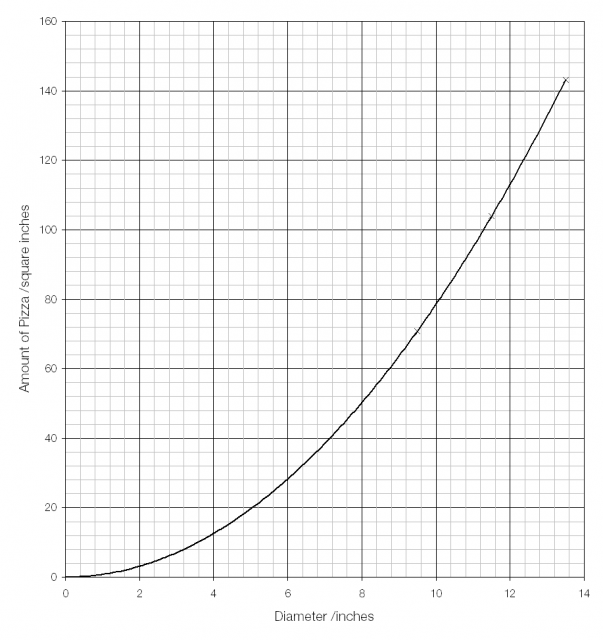Looking at the cost per square inch shows an interesting pattern: as size increases, the cost per square inch goes down. (Data from Dominos Pizza.)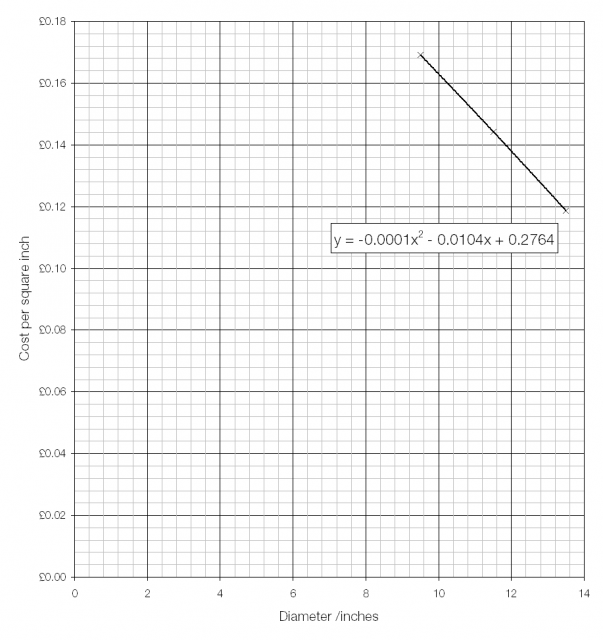If we take the trend that the data is following and solve the equation of the trendline (line of best fit) then we find two possible solutions: the cost per square inch falls to £0.00 at a diameter of either −128 inches or 22 inches. Since a pizza with a diameter of negative 128 inches is clearly ridiculous, it’s the free 22-inch pizza that is interesting.# Shapes of equal width

Circular objects roll because a circle is a shape of equal width. No matter where you measure from, the distance from one side to the other (through the centre) is the same. But circles aren’t the only shape with this property.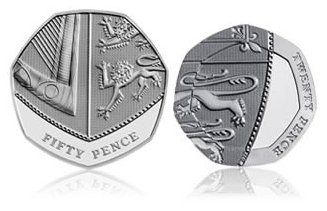Two British coins are shapes of equal width: the 20p (21.4 mm) and 50p (27.3 mm) coin. Having an equal width makes the coins able to roll and prevents them from getting stuck in machinery. Shapes of equal width also make good manhole covers; because no “side” is any shorter than any other, it is impossible for the cover to fall down the manhole. Circles are easiest to make which is why most manhole covers are circular.# Tissot’s indicatrix

Flat two-dimensional maps are unable to accurately represent the curved three-dimensional surface of the Earth; some distortion of area, shape, distance and/or scale is unavoidable. Tissot’s indicatrix, invented by French mathematician and cartographer Nicolas Tissot, is a method for assessing and indicating the amount of distortion present in a map.

The indicatrix is created by placing an array of tiny circles on the Earth’s surface and then mapping those circles along with the rest of the Earth’s features; the size of the circles is then increased to make them visible.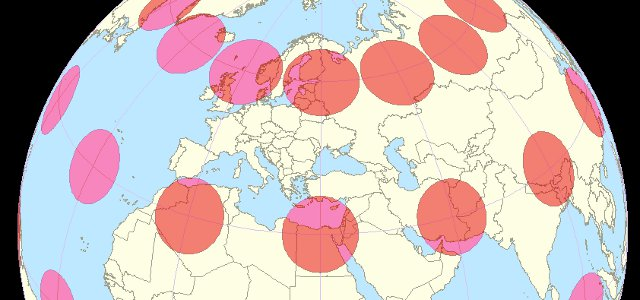The Mercator projection maintains correct shapes but exaggerates the size of land masses as distance from the equator increases. On the Mercator projection Greenland appears to have a similar area as Africa, despite being only 7% of the size in reality; it also makes Antartica look much larger than it is.*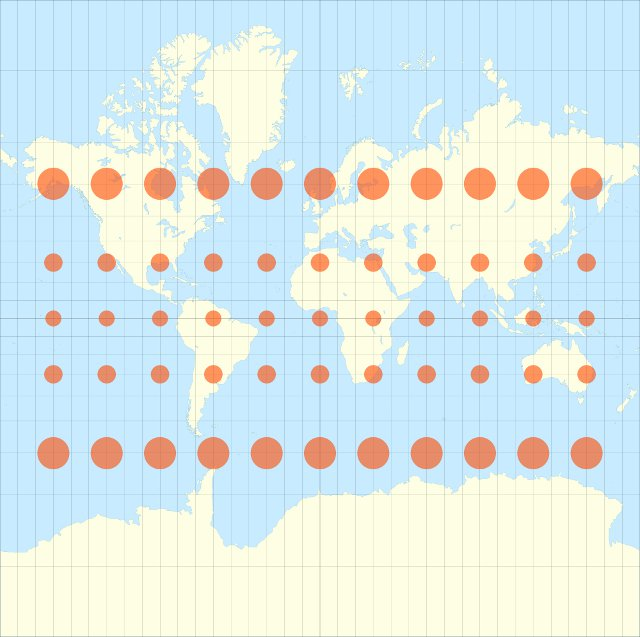The equirectangular projection preserves approximate size at the expense of shape: land masses away from the equator are distorted both horizontally and vertically but distances along meridians (lines of longitude) are preserved.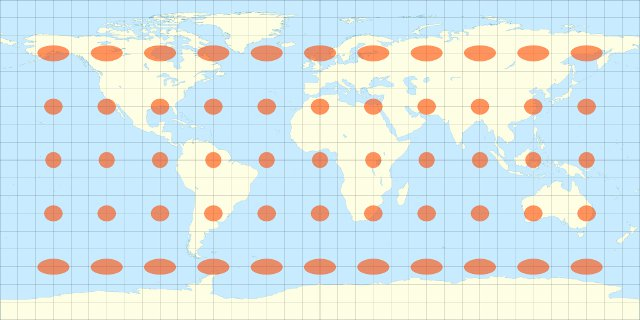The use of rectangular projections for whole world maps is now widely discouraged; they are considered suitable only for small areas where any distortion will be negligible.

The non-rectangular Robinson and Winkel tripel projections both attempt to find an acceptable compromise between distortions of shape and distance.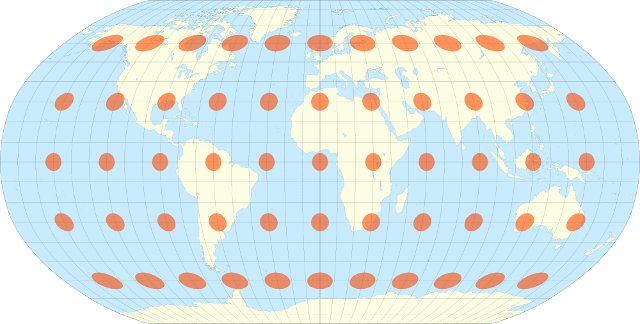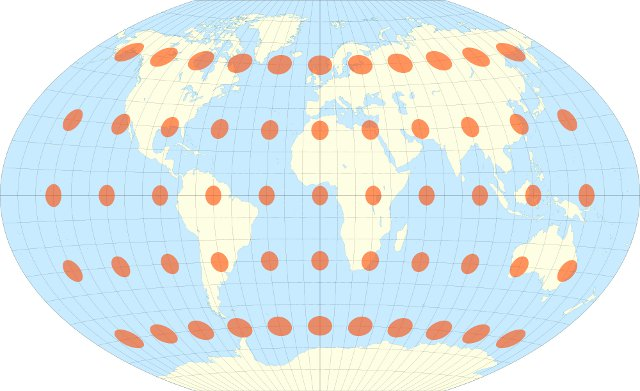* The Mercator projection’s early popularity was due to its heavy use by sailors – on a Mercator map a constant heading yields a straight line.